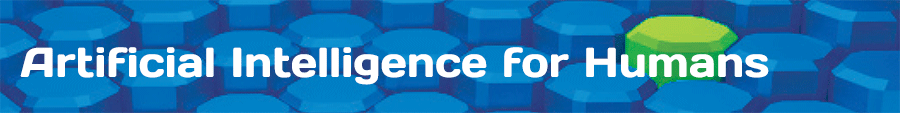Volume 3: Deep Learning and Neural Networks

• Chapter 1: Neural Network Basics
• Chapter 2: Self Organizing Maps
• Chapter 3: Hopfield & Boltzmann Machines
• Chapter 4: Feedforward Neural Networks
• Chapter 5: Training & Evaluation
• Chapter 6: Backpropagation Training
• Chapter 7: Other Propagation Training
• Chapter 8: NEAT, CPPN and HyperNEAT
• Chapter 9: Deep Learning
• Chapter 10: Convolutional Neural Networks
• Chapter 11: Pruning and Model Selection
• Chapter 12: Dropout and Regularization
• Chapter 13: Time Series and Recurrent Networks
• Chapter 14: Architecting Neural Networks
• Chapter 15: Visualization
• Chapter 16: Modeling with Neural Networks